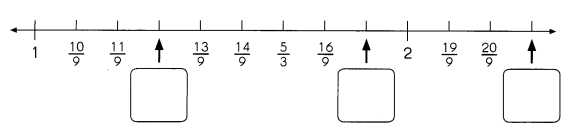# Math in Focus Grade 4 Chapter 6 Practice 4 Answer Key Improper Fractions

Practice the problems of Math in Focus Grade 4 Workbook Answer Key Chapter 6 Practice 4 Improper Fractions to score better marks in the exam.

## Math in Focus Grade 4 Chapter 6 Practice 4 Answer Key Improper Fractions

Write each mixed number as an improper fraction.

Example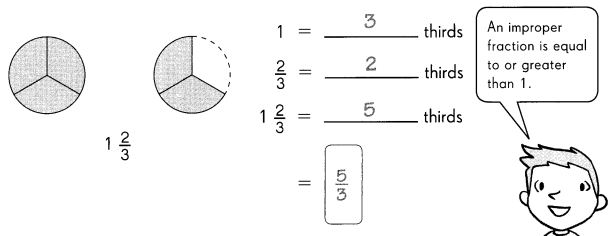Question 1.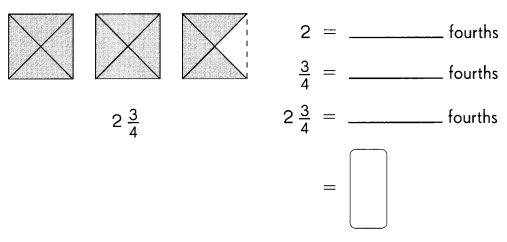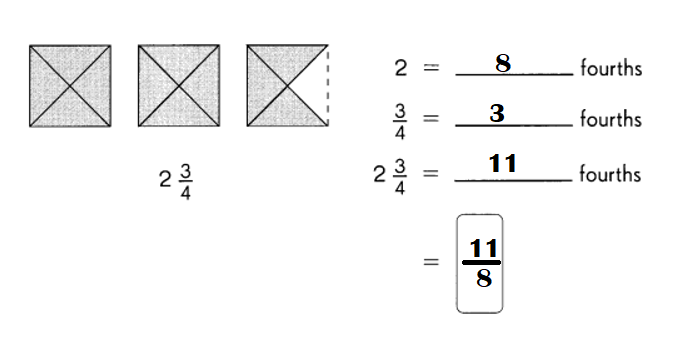Explanation:
2 = 8 fourths.
$$\frac{3}{4}$$ = 3 fourths.
2$$\frac{3}{4}$$ = 11 fourths.

Question 2.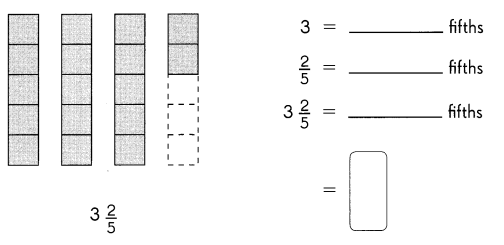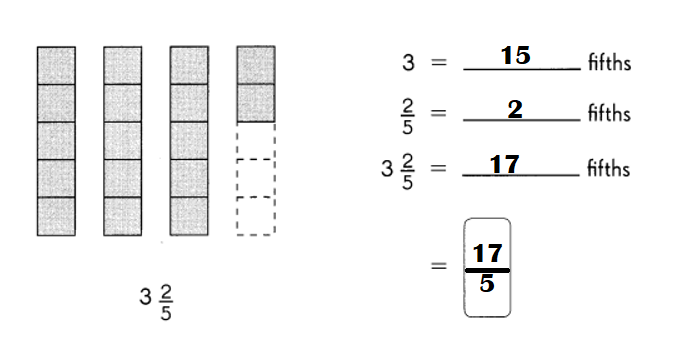Explanation:
3 = 15 fifths.
$$\frac{2}{5}$$ = 2 fifths.
3$$\frac{2}{5}$$ = 17 fifths.

Write the improper fractions for the shaded parts
Question 3.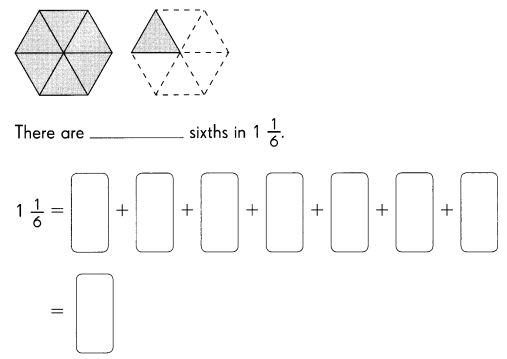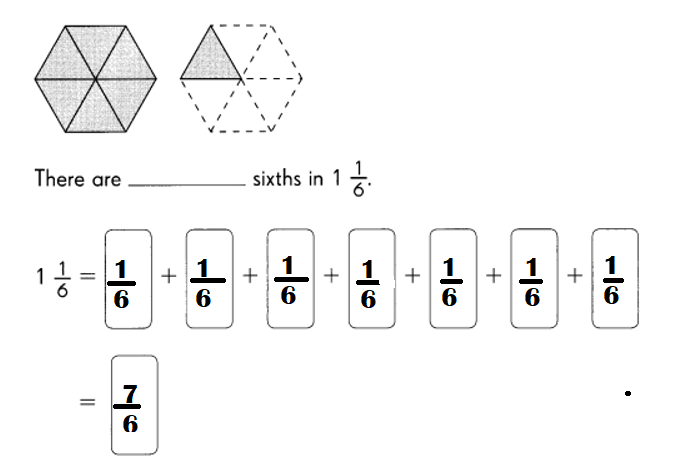Explanation:
1 = 6 sixths.
= 1 + $$\frac{1}{6}$$
= $$\frac{7}{6}$$

Question 4.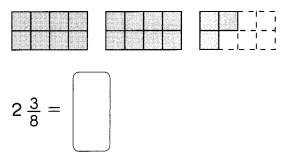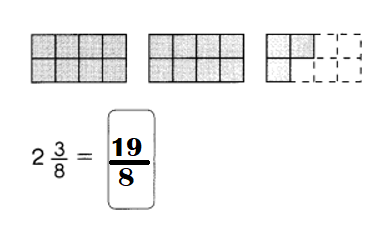Explanation:
1 = 8 eighths.
= 2 + $$\frac{3}{8}$$ = 2$$\frac{3}{8}$$ .

Question 5.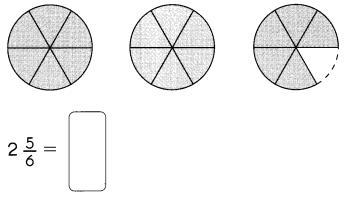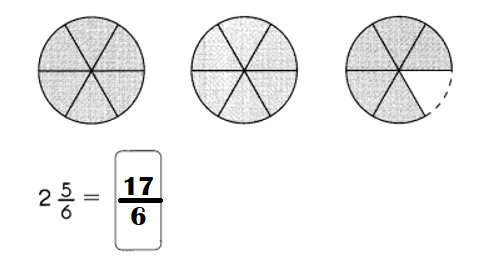Explanation:
1 = 6 sixths.
= 2 + $$\frac{5}{6}$$
= 2$$\frac{5}{6}$$

Write the improper fraction for the shaded parts.

Question 6.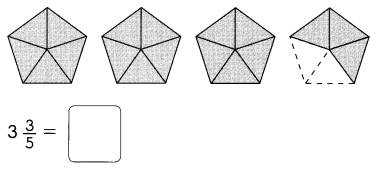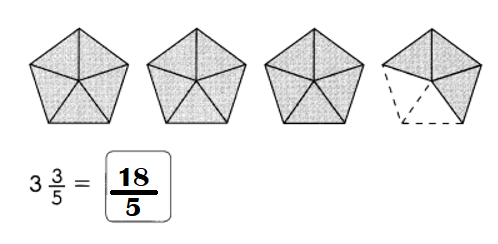Explanation:
3$$\frac{3}{5}$$ = (15 + 3) ÷ 5
= $$\frac{18}{5}$$

Write a mixed number and an improper fraction for each model.
Example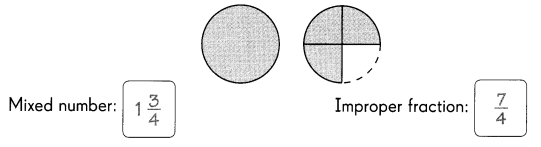Question 7.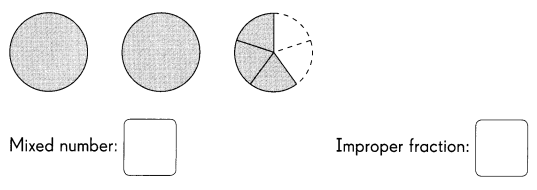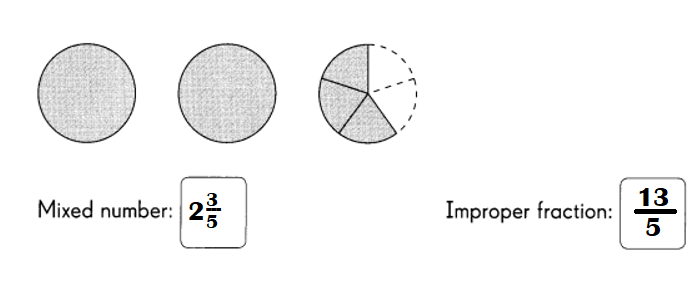Explanation:
Mixed number = 2 + $$\frac{3}{5}$$
= 2$$\frac{3}{5}$$.
Improper fraction = 1 + 1 + $$\frac{3}{5}$$
= 2 + $$\frac{3}{5}$$
= (10 + 3) ÷ 5
= $$\frac{13}{5}$$ or 13 ÷ 5.

Question 8.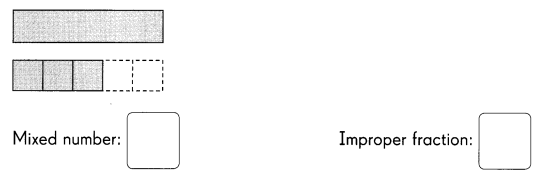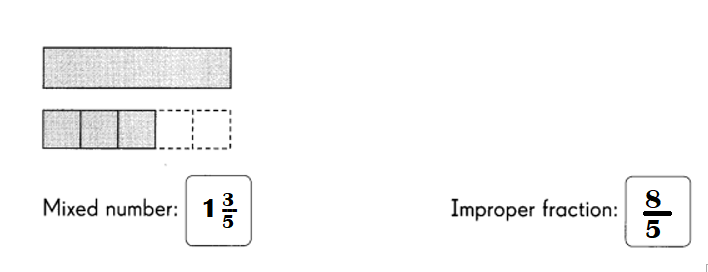Explanation:
Mixed number = 1 + $$\frac{3}{5}$$
= 1$$\frac{3}{5}$$.
Improper fraction =  1 + $$\frac{3}{5}$$
= (5 + 3) ÷ 5
= $$\frac{8}{5}$$ or 8 ÷ 5.

Write a mixed number and an improper fraction for each model.
Question 9.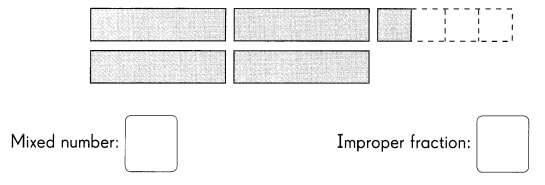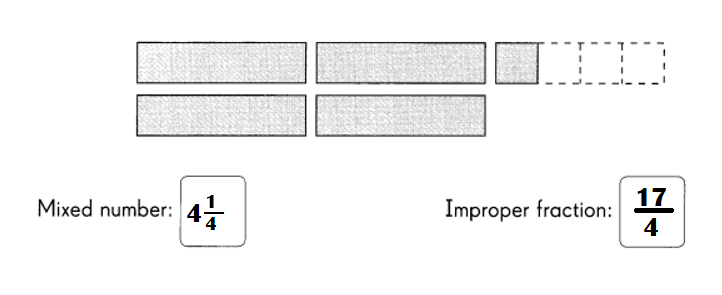Explanation:
Mixed number = 1 + 1 + 1 + 1 + $$\frac{1}{4}$$
= 4 + $$\frac{1}{4}$$
= 4 $$\frac{1}{4}$$.
Improper fraction = 1 + 1 + 1 + 1 + $$\frac{1}{4}$$
= 4 + $$\frac{1}{4}$$
= (16 + 1) ÷ 4
= $$\frac{17}{4}$$ or 17 ÷ 4.

Write the missing improper fraction in each box. Express the answers in simplest form.
Question 10.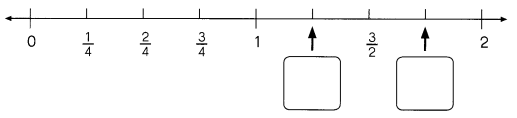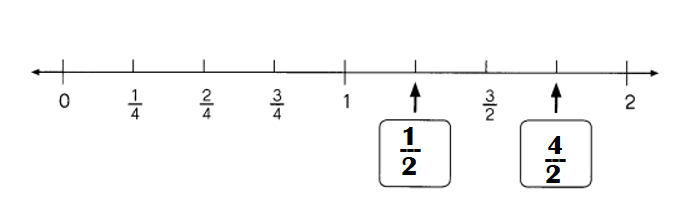Explanation:
$$\frac{3}{2}$$ – 1 = (3 – 2) ÷ 2
= 1 ÷ 2 or $$\frac{1}{2}$$

= $$\frac{3}{2}$$ + $$\frac{1}{2}$$
= (3 + 1) ÷ 2
= 4 ÷ 2 or $$\frac{4}{2}$$

Question 11.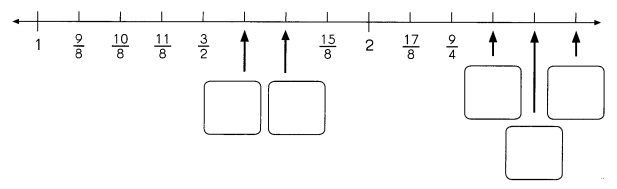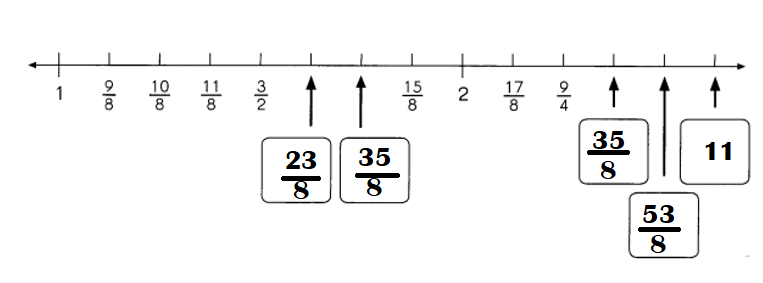Explanation:
$$\frac{11}{8}$$ + $$\frac{3}{2}$$ = (11 + 12) ÷ 8
= 23 ÷ 8 or $$\frac{23}{8}$$
$$\frac{3}{2}$$ + $$\frac{23}{8}$$ = (12 + 23) ÷ 8
= 35 ÷ 8 or $$\frac{35}{8}$$
$$\frac{17}{8}$$ + $$\frac{9}{4}$$ = (17 + 18) ÷ 8
= 35 ÷ 8 or $$\frac{35}{8}$$
$$\frac{9}{4}$$ + $$\frac{35}{8}$$ = (18 + 35) ÷ 8
= 53 ÷ 8 or $$\frac{53}{8}$$
$$\frac{35}{8}$$ + $$\frac{53}{8}$$ = (35 + 53) ÷ 8
= 88 ÷ 8
= 11.

Write each improper fraction in a box to show its correct location on the number line.
Question 12.
$$\frac{4}{3}$$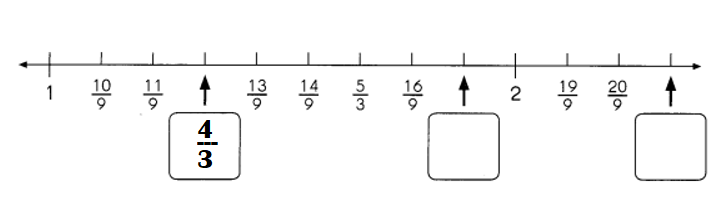Explanation:
$$\frac{4}{3}$$ = 1.33.
On number line = $$\frac{12}{9}$$ = 1.33.

Question 13.
$$\frac{7}{3}$$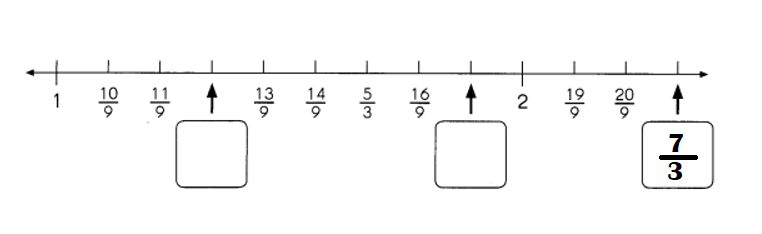Explanation:
$$\frac{7}{3}$$ = 2.33.
On number line = $$\frac{21}{9}$$= 2.33.

Question 14.
$$\frac{17}{9}$$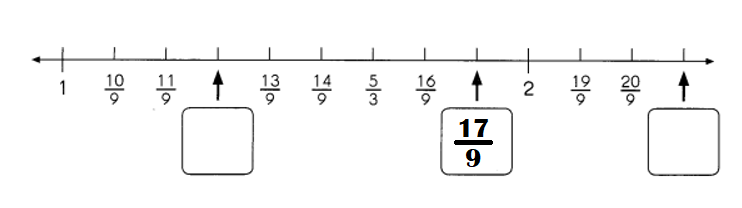$$\frac{17}{9}$$ = 1.89.
On number line = $$\frac{17}{9}$$ = 1.89.